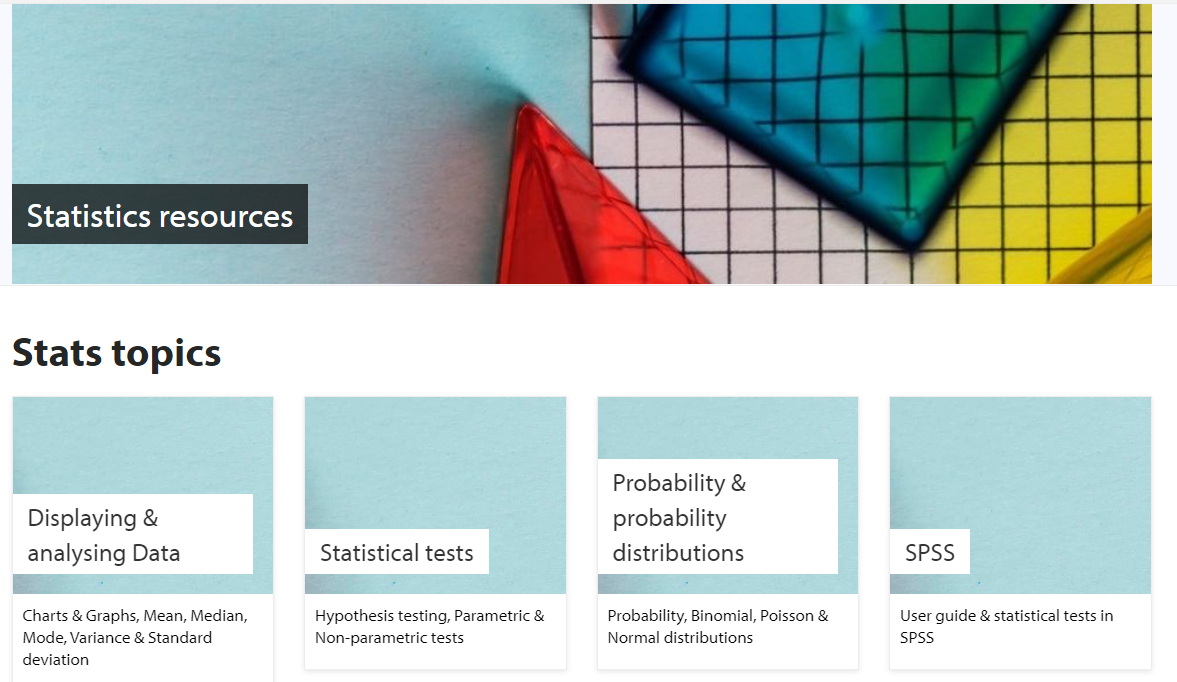We appreciate that many students will be requiring assistance with the statistics and data for their MSc Professional Project Dissertation, and it can sometimes be difficult to find the most efficient help required. However, we highly advise our students to make the most of the following resources, hosted by USW:

As you will see in the visual above, the statistics resources are particularly useful in the following statistical topics:

• Displaying and Analysing Data
• Statistical Tests
• Probability and Probability Distributions
• SPSS Software (inlcuding an SPSS Guide and Tests in SPSS)

The university also reccommends several websites, such as those of the below:

Statstutor - university level statistics support

Stat trek - online tutorials covering statistics, probability and survey sampling

HELM Workbooks - Learning materials on a wide range of maths & stats topics

Statistics lectures - short videos on statistics

Wolfram alpha - ask almost any maths or stats question and get a detailed answer